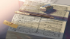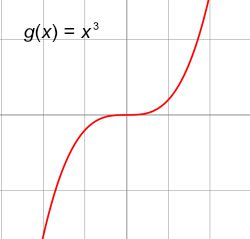Discussion about math, puzzles, games and fun.   Useful symbols: ÷ × ½ √ ∞ ≠ ≤ ≥ ≈ ⇒ ± ∈ Δ θ ∴ ∑ ∫  π  -¹ ² ³ °

You are not logged in.

## #1 2018-02-02 05:45:28

Hannibal lecter
MemberRegistered: 2016-02-11
Posts: 225
Website

### a montonic funtion

Hi,

what is the explanation of  1) "  A function f(x) is monotonic if it increases for all x or decreases for all x.?

what is the meaning of monotonic ?

I know what is the increasing function and what is the decreasing

but what is the meaning of monotonic

and what is

2) Strictly Decreasing?
3) Strictly Increasing?

what is the meaning of Strictly ?????

Last edited by Hannibal lecter (2018-02-02 05:53:42)

Wisdom is a tree which grows in the heart and fruits on the tongue

Offline

## #2 2018-02-02 08:21:19

ganesh
AdministratorRegistered: 2005-06-28
Posts: 27,849

### Re: a montonic funtion

Hi Hannibal lecter,

Please see Increasing and Decreasing Functions.

A monotonic function is a function which is either entirely nonincreasing or nondecreasing. A function is monotonic if its first derivative (which need not be continuous) does not change sign.

Monotonicity

The monotonicity of a function tells us if the function is increasing or decreasing. A function is increasing when its graph rises from left to right. In technical terms, a function is increasing on an interval I if for any x1 and x2 in I, x1 is less than x2 implies that f(x1) is less than f(x2). Basically, this says that when x1 is less than x2, then the function evaluated at x1 is less than the function evaluated at x2.

A function is decreasing when its graph falls from left to right. Again, the technical definition says that a function is decreasing on an interval I if for any x1 and x2 in I, x1 is less than x2 implies that f(x1) is greater than f(x2). In other words, a function is decreasing on an interval I when it is the case that whenever x1 is less than x2, f(x1) is greater than f(x2).

The monotonicity of a function is directly related to the function's derivative. A function is increasing when its derivative is positive, and a function is decreasing when its derivative is negative. For example, consider the function f(x) = x^2. The derivative is f ' (x) equals 2x. Now consider where 2x is positive and negative.

2x is greater than 0.
Divide both sides by 2.
x is greater than 0.

Therefore, f is positive when x is greater than 0, so f is increasing on the interval x is greater than 0.

Similarly, consider where 2x is negative:

2x is less than 0
Divide both sides by 2
x is less than 0

Thus, f ' is negative when x is less than 0, and f is decreasing on the interval x is less than 0.

Montonic Function

When a function is increasing on its entire domain or decreasing on its entire domain, we say that the function is strictly monotonic, and we call it a monotonic function. For example, consider the function g(x) equals x^3:

Notice the graph of g is increasing everywhere. Therefore, this is a monotonic function. We can also observe this by looking at the derivative of g. The derivative of g(x) equals x^3 is g' (x) equals 3x^2. Notice that no matter what we plug in for x in the derivative, it gets squared, making it positive. Thus, the derivative is always positive, so g is always increasing. Therefore, g is a monotonic function.

Non-Monotonic Function

To solidify our understanding of what a monotonic function is, let's consider what a non-monotonic function is. A non-monotonic function is a function that is increasing and decreasing on different intervals of its domain.

For example, consider our initial example f(x) equals x^2. We saw that this function is increasing on the interval x is greater than 0, and decreasing on the interval x is less than 0. Since the function is increasing and decreasing on different intervals of its domain, the function is a non-monotonic function. Basically, if a function is not increasing on its entire domain or decreasing on its entire domain, then the function is not monotonic, and we say that it is non-monotonic.

Monotonic Function :Strictly increasing function : a function having the property that for any two points in the domain such that one is larger than the other, the image of the larger point is greater than the image of the smaller point.

Strictly decreasing function : a function having the property that for any two points in the domain such that one is larger than the other, the image of the larger point is less than the image of the smaller point.

It is no good to try to stop knowledge from going forward. Ignorance is never better than knowledge - Enrico Fermi.

Nothing is better than reading and gaining more and more knowledge - Stephen William Hawking.

Offline

## #3 2018-02-02 09:01:37

Hannibal lecter
MemberRegistered: 2016-02-11
Posts: 225
Website

### Re: a montonic funtion

thanks,

but about the Strictly increasing/decreasing
I didn't understand, can you provide for me an examples?

Wisdom is a tree which grows in the heart and fruits on the tongue

Offline

## #4 2018-02-02 12:03:41

ganesh
AdministratorRegistered: 2005-06-28
Posts: 27,849

### Re: a montonic funtion

Hi,

Hope these help.

Find the intervals in which the function f is given by

is
(i) strictly increasing
(ii) strictly decreasing

Solution : Step 1 : Calculating f'(x)

f'(x) = 4x - 3

Step 2 : Put f'(x) = 0
4x - 3 = 0.
4x = 3.
x = 3/4.

Step 3 : Point 3/4 divides the real line into disjoint intervals

and

Hence, f is strictly increasing in

and f is strictly decreasing in
.

It is no good to try to stop knowledge from going forward. Ignorance is never better than knowledge - Enrico Fermi.

Nothing is better than reading and gaining more and more knowledge - Stephen William Hawking.

Offline

## #5 2018-02-02 20:22:52

zetafunc
ModeratorRegistered: 2014-05-21
Posts: 2,231
Website

### Re: a montonic funtion

Hannibal lecter wrote:

Hi,

what is the explanation of  1) "  A function f(x) is monotonic if it increases for all x or decreases for all x.?

what is the meaning of monotonic ?

I know what is the increasing function and what is the decreasing

but what is the meaning of monotonic

and what is

2) Strictly Decreasing?
3) Strictly Increasing?

what is the meaning of Strictly ?????

First, let's examine what is meant by the word 'increasing'. How can you look at a graph and immediately tell if it is increasing or not? One way is to look at the slope (or, if you've done any calculus, the 'derivative', which tells you the slope of the function at every point). If the slope at that point is positive, then the function is increasing. If it is negative, it is decreasing. If it is 0, then it's stationary. Now let's try to understand what is meant by 'monotone'.This is an example of a function which is monotonically increasing. Notice that the graph is always 'heading upwards' (think about the tangents to this curve for a more accurate description). This happens until we get to the middle, where the graph resembles a horizontal line. It's not increasing along this part -- but it also isn't decreasing. In other words, if something is monotonically increasing, then it doesn't decrease.This is an example of a function which is monotonically decreasing. Notice that the graph is always 'heading downwards', except when we get to the horizontal part of the curve. In other words, if something is monotonically decreasing, then it doesn't increase.That's a function which isn't monotone at all -- do you see why?

As for 'strictly increasing', then this means that the function must always be increasing -- it won't have any 'horizontal parts'. Likewise for 'strictly decreasing'.

There are formal mathematical definitions for all of these terms, by the way -- but I want to make sure that you understand what the picture looks like first. Does this help?

Offline

## #6 2018-02-03 00:12:12

Hannibal lecter
MemberRegistered: 2016-02-11
Posts: 225
Website

### Re: a montonic funtion

yes that's help me too much I understand now what is the meaning of all of this

but I want to know more about the conditions ?

when x1 < x2 then f(x1) ≥ f(x2)         Decreasing
when x1 < x2 then f(x1) > f(x2)         Strictly Decreasing

and the rest of the other condition of monotonic and increasing
why I can't understand this conditions while I have an understanding of increasing/decreasing function ?

can you provide to me an explanation for these conditions please and how to solve a specific exercise

Wisdom is a tree which grows in the heart and fruits on the tongue

Offline

## #7 2018-02-03 00:22:58

zetafunc
ModeratorRegistered: 2014-05-21
Posts: 2,231
Website

### Re: a montonic funtion

Those inequalities are essentially saying everything that we've just discussed: imagine that
and
are points on the
-axis. In fact, let's use
and
instead.

We know that
is to the right of
on the
-axis, i.e.
. Then,
is a point on the curve, and
is a point on the curve. So if
, this means that the point corresponding to
is 'below' the point corresponding to
.

The statement
just says that for any two different points you pick, one of them is always going to be 'below' the other -- which means that it's strictly increasing.

The difference between 'strictly increasing' and 'monotonically increasing' is virtually the same as the difference between '
' and '
' -- make sense?

Offline

## #8 2018-02-03 00:30:34

Hannibal lecter
MemberRegistered: 2016-02-11
Posts: 225
Website

### Re: a montonic funtion

is there a full example of the strictly increasing that you mention with number 7 and 3 please? and with a graph please

abut the monotonically increasing, if it's the same why we put ≤  ? what is that mean

Last edited by Hannibal lecter (2018-02-03 00:31:49)

Wisdom is a tree which grows in the heart and fruits on the tongue

Offline

## #9 2018-02-03 00:33:11

zetafunc
ModeratorRegistered: 2014-05-21
Posts: 2,231
Website

### Re: a montonic funtion

OK, take the line
. Do you see that
?

The '≤' sign accounts for the fact that the function doesn't have to be increasing at all points.

Offline

## #10 2018-02-03 01:20:16

Hannibal lecter
MemberRegistered: 2016-02-11
Posts: 225
Website

### Re: a montonic funtion

I see thanks a lot

Wisdom is a tree which grows in the heart and fruits on the tongue

Offline

## Board footer

Powered by FluxBB